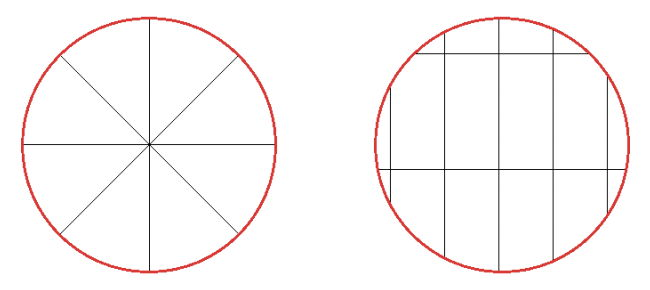시간 제한 메모리 제한 제출 정답 맞은 사람 정답 비율
2 초 512 MB 0 0 0 0.000%

문제

Pizzas are usually cooked as a circle, and are often cut “triangularly” along lines moving out from the center, as shown on the left in Figure D.1 below. However, some heretical people prefer that the pizza be cut into rectangular pieces, as shown on the right in the figure:Figure D.1: Sample pizza cuts. The pizza on the right corresponds to Sample Inputs 1 and 2.

As you can see, besides the obvious aesthetic issues involved, the pieces that intersect the boundary of the circle may not be complete rectangles. Sometimes these pieces are close to the largest size piece, but other times they are so small as to be useless. Given a pizza radius, lengths and widths of the rectangular cuts, and a “too small” percentage (for example, any slice that is less than 50% of the largest slice is too small), how many slices are cut that will be “too small”?

입력

Input consists of a single line containing six numbers r dx dy x y p, where r (1 ≤ r ≤ 1 000) is the radius of the pizza, dx and dy (1 ≤ dx, dy ≤ 3 000) are the width and height of the pizza cuts, x and y (−10 000 ≤ x, y ≤ 10 000) is the intersection point of one vertical and one horizontal cut, and p (0.0 < p < 1.0) is the percentage defining a “too small” piece. All values are integers except for p, which is a real number with at most 3 decimal places. The pizza is centered at point (0, 0).

출력

Display the number of pieces of pizza that are less than p times the size of the largest piece. A piece with area X is considered p times smaller than another piece with area Y if |X/Y - p |≤ 10−6.

예제 입력 1

100 45 90 0 -20 0.1

4

예제 입력 2

100 45 90 0 -20 .999

14